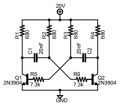# Astable Multivibrator not switching

#### MacIntoshCZ

Joined Sep 4, 2019
7
I am having problems with circuit, that I designed.R1=R2=R3=R4= 680 ohm; C1=C2 = 20nF; R5=R6 = 7,3kohm Circuit works only when voltage is about 0,5-0,7V and resistors r5/r6 are not connected. It "should" oscillate at 50Khz.

** R5=R6 Are calculated for hfe=306 measured. Designed for 20V -> 680 ohm -> U/R -> 20/680 = 0,0294A. Thats what transistor should turn on. With hfe 306 -> 0,0294/306 -> 0,000096 A. Thats minimal current for turn it ON. So to bypass turning second transistor On when capacitor rise 0,7V - resistor is calculated for 20V -> 20/0,000096 = 208kOhms. With resistors connected, there is no switching and i got nice smell from burning resistor R1 when rising voltage to 20V.
Problem is not resistors. I can change them later. Does abyone know why its not oscilating?
Thanks very much

#### AlbertHall

Joined Jun 4, 2014
10,046
20V across 680Ω is 0.58W. What rating resistors are R1 and R4.
Next make R5 and R6 zero ohms, and R2, R3 100k and see what happens.

#### MacIntoshCZ

Joined Sep 4, 2019
7
20V across 680Ω is 0.58W. What rating resistors are R1 and R4.
Next make R5 and R6 zero ohms, and R2, R3 100k and see what happens.
Its 250mw but it should oscillate at 50% duty.
If r5 and r6 are 0 ohms then it oscillate only when vcc is 0,5-0,9 Volts. And it looks similary like this: (not mine photo)
+ If I rise voltage above 1volt transistor Q1 is always on
+ If i Change R2 and R3 to 100k then it will change oscilating frequency from 50khz. or it Wont?Last edited:

#### AlbertHall

Joined Jun 4, 2014
10,046
It will change the frequency - but at least it should oscillate. Then you can select the capacitors to get the frequency you want.

#### LesJones

Joined Jan 8, 2017
2,666
Try replacing R5 and R6 with a diode such as a 1N4148 with the cathode end of the diode to the base. This will allow the junction of R3 and C1 (And R2 and C2.) to go down to - 20 volts without being clamped by the reverse base emitter junction breakdown voltage. Exceeding this breakdown voltage could also damage the transistors.

Les.

•AlbertHall and bertus

#### RPLaJeunesse

Joined Jul 29, 2018
104
Issues I see: The base-emitter junction doesn't want to see more than about -4v. With a 20v supply you will greatly exceed this. Add reverse diodes across each base-emitter to clamp the bases at -0.7v, and do not delete R5 and R6. While hfe measures 306 don't design around that, especially since you want saturated switching. At 50mA collector current the 2N3904 is rated for a minimum hfe of 60, use that gain to determine base drive for your 680 Ohm collector loads. I calculate that the sum of (R2 + R5) (or R3 + R6) needs to be about 40K and, thinking R5 should be around 10x R2, would use 33K for R5/R6 and 6.8K for R2/R3.

#### Bordodynov

Joined May 20, 2015
2,628
Roughly speaking, you've taken a very bad ratio of resistors in bases and collectors.

•RPLaJeunesse

#### dl324

Joined Mar 30, 2015
10,984
I am having problems with circuit, that I designed.
This is what your schematic would look like if you drew it more conventionally and added component values:R5 and R6 are unnecessary and the values for R1-4 and C1-2 are too low. The transistors are being used as switches and you should be using a beta 0f 10, not 306 (which is above the maximum specified from Fairchild).